# Test: Skew Lines

## 10 Questions MCQ Test Mathematics (Maths) Class 12 | Test: Skew Lines

Description
This mock test of Test: Skew Lines for JEE helps you for every JEE entrance exam. This contains 10 Multiple Choice Questions for JEE Test: Skew Lines (mcq) to study with solutions a complete question bank. The solved questions answers in this Test: Skew Lines quiz give you a good mix of easy questions and tough questions. JEE students definitely take this Test: Skew Lines exercise for a better result in the exam. You can find other Test: Skew Lines extra questions, long questions & short questions for JEE on EduRev as well by searching above.
QUESTION: 1

Solution:
QUESTION: 2

### For which value of a lines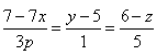and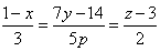are perpendicular?

Solution:

(x-1)/(-3) = (y-2)/(2p/7) = (z-3)/2
(x-1)(-3p/7) = (y - 5)/1 = (z - 6)/(-5)
The direction ratio of the line are -3, 2p/7, -2 and (-3p)/7, 1, -5
Two lines with direction ratios, a1, b1, c1 and a2, b2, c2 are perpendicular to each other if a1a2 + b1b2 + c1c2 = 0
Therefore, (-3)(-3p/7) + (2p/7)(1) + 2(-5) = 0
(9p/7) + (2p/7) = 10
11p = 70
p = 70/1

QUESTION: 3

### The shortest distance between the lines whose equations are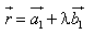and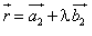is:

Solution:
QUESTION: 4

Two lines whose direction ratios are a1,b1,c1 and a2,b2,c2 are perpendicular, if

Solution:
QUESTION: 5

The shortest distance between the parallel lines whose equations are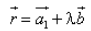and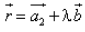Solution:
QUESTION: 6

The angle between the pair of lines given by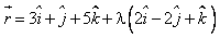and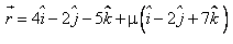is:

Solution:
QUESTION: 7

The angle between the lines x = 2y = – 3z and – 4x = 6y = – z is:​

Solution:

x = 2y = -3z     -4x = 6y = -z
x/1 = y/(½) = z(-⅓)                   x/(-¼) = y/(⅙) = z/(-1)
Cosθ = [(a1a2 + b1b2 + c1c2)/(a1 + b1 + c1)½ * (a2 + b2 + c2)½]
Cosθ ={[(1*(-¼)) + (½)(⅙) + (-⅓)(-1)]/[(1)2 + (½)2 + (-⅓)2]1/2 * [(-¼)2 + (⅙)2 + (-1)2]1/2}
= {[(-¼ + 1/12  - ⅓)]/[2 + 1 - ⅔]1/2 * [ -½ + ⅓ -½]½}
Cosθ = 0
θ = 90deg

QUESTION: 8

The angle between the lines whose direction cosines are given by the equations 3l + m + 5n = 0, 6nm - 2nl + 5lm = 0 is:

Solution:

3l + m + 5n = 0
m = - (3l + 5n) -----------(1)
6mn - 2nl + 5lm = 0 ----------(2)
Substitute m=-(3l+5n) in eq(2)
⇒ 6[- (3l + 5n)]n - 2nl + 5l[- (3l + 5n)] = 0
⇒( -18ln - 30n)n-2nl-15l^2+25ln=0
⇒ l(l + 2n) + n(l + 2n) = 0
⇒ (l + n) (l + 2n) = 0
∴ l = - n and l = -2n
( l / -1 ) = ( n / 1) and ( l / -2) = ( n / 1) -------(3)
Substitute l in equation 1, we get
m = - (3l + 5n)
m = -2n and m = n
( m / -2) = ( n / 1) and ( m / 1) = ( n / 1 ) --------(4)
From ( 3) and (4) we get
( l / -1 ) = ( m / -2) = ( n / 1),
( l / -2) = ( m / 1) = ( n / 1 )
l : m : n = -1 : -2 : 1
l : m : n = -2 : 1 : 1
i.e D.r's ( -1, -2, 1) and ( -2 , 1 , 1)
Angle between the lines whose direction cosines are
Cos θ = ( -1 × -2 + -2×1 + 1×1) / √ ((-1)^2+(-2)2+12))*√((-2)2+12+12))
Cos θ = 1 / √6 √6
Cos θ = 1 / 6
∴ θ = cos inverse of (1/6)
∴Angle between the lines whose direction cosines is cos-1(1/6)

QUESTION: 9

The angle between the lines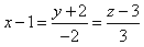and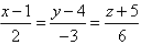is:

Solution:
QUESTION: 10

The length of the shortest distance between the lines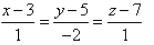and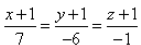is:

Solution:

let P and Q be the points on the given lines, respectively. then the general coordinates of P and Q are:
P(k+3, -2k+5, k+7) and Q (7m-1, -6m-1, m-1)
therefore the direction ratios of PQ are (7m-k-4,-6m+2k-6, m-k-8)
now PQ will be the shortest distance if it is perpendicular to both the given lines, therefore by the condition of perpendicularity,
1(7m-k-4) -2(-6m+2k-6) + 1(m-k-8) = 0  (1)
7(7m-k-4) -6(-6m+2k-6) + 1(m-k-8) = 0  (1)
now solving (1) and (2),
m=0 and k = 0
hence the points are P(3,5,7) and Q (-1,-1,-1), therefore the shortest distance between the lines
PQ = sqrt((3+1)2+(5+1)2 +(7+1)2
= sqrt(16+36+64) = sqrt(116)
= 2sqrt(29)